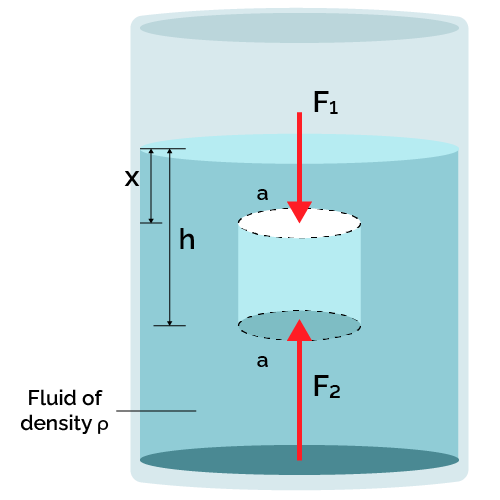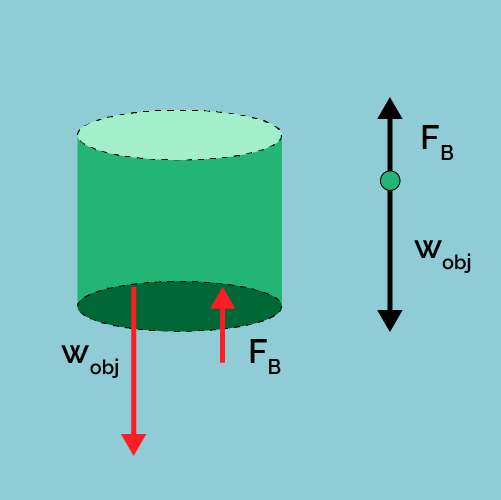# Archimedes' Principle Calculator

Created by Purnima Singh, PhD
Reviewed by Steven Wooding
Last updated: Apr 29, 2022

Omni's Archimedes' principle calculator helps you understand the concept of buoyancy. You can also use it to determine objects' densities and changes in mass and weight when they are submerged in a fluid.

Have you ever wondered why certain objects float and others sink? If you have, you have come to the right place.

Continue reading this article to learn about Archimedes' principle and his formula. You will also find examples of buoyancy force calculations and some interesting applications of Archimedes' principle.

Let us first start with learning what Archimedes' principle is.

## What is the Archimedes' principle? – Archimedes' principle definition

When we immerse an object in any fluid, there is a net upward force on the object. This upward force is called the buoyant force or buoyancy.

The origin of the buoyant force lies in the fact that the pressure increases with an increase in depth in a fluid (remember the hydrostatic pressure equation). Therefore, the upward force applied by the fluid to the bottom of the object is greater than the downward force applied to the top of the object. Check out our dedicated tool to learn more about buoyancy and perform buoyancy experiments at home.

According to Archimedes' principle, when an object is partially or wholly immersed in a fluid, it displaces some of the fluid. The buoyant force acting on the object is equal to the weight of the fluid displaced by it.

To understand what the principle means, let us try to derive a formula for the Archimedes principle.

## Archimedes' principle formula

Let us consider an object of height $h-x$ and mass $M$ immersed in a fluid of density $\rho$ (see figure 1). If $a$ is the cross-sectional area of its top and bottom face, we can write expressions for the vertically downward ($F_1$) and upward force ($F_2$) on the object as:Fig 1: Pressure on an object immersed in a fluid increases with depth.
\small \begin{align*} F_1 &= p_1 a = x \rho g a\\ F_2 &= p_2 a = h \rho g a \end{align*}

where:

• $p_1 = x \rho g$ – Pressure on the top face of the object;
• $p_2 = h \rho g$ – Pressure on the bottom face of the object;
• $x$ – Depth of the top surface of the object in the fluid; and
• $h$ – Depth of the bottom surface of the object in the fluid.

Since $h>x$, we note that $F_2 > F_1$. The net upward force or buoyant force acting on the object is:

\small \begin{align*} F_B & = F_2 - F_1\\ & = (h -x) \rho g a\\ & = [(h-x)\times a]\rho g\\ & = V\rho g \end{align*}

Where $V$ is the volume of the object, and it is equal to the volume of the displaced fluid $V_{fl}$.

If $m$ is the mass of the displaced fluid, we can rewrite the above equation as:

\small \begin{align*} F_B & = V_{fl} \rho g \\ & = m g \end{align*}

In other words, the buoyant force acting on the object is equal to the weight of the fluid displaced by the object.

## Buoyancy and the law of floatation

Since the true weight of the object is $W_{obj} = M g$, and the upward force on it is $F_B= m g$, the observed weight of the object in the fluid is (see figure 2):Fig 2: Buoyant force experienced by an object immersed in a fluid.
\small \begin{align*} W_{obs} &= W_{obj} - F_B\\ &= Mg - m g \end{align*}

This means that when an object is immersed in a fluid, its observed weight becomes less than its true weight by an amount equal to the weight of the fluid displaced by the object.

Now, if the weight of the fluid displaced by the immersed body is greater than or equal to the body's weight, the body will float. Otherwise, it will sink. This is also known as the law of floatation.

Another fact that follows from the law mentioned above is that if the average density of an object is less than that of the fluid it is immersed in, it will float. In contrast, objects with an average density greater than the fluid will sink.

## Buoyancy force calculation example

Now, as an example, let us calculate the buoyant force on a $1.00 \times 10^6 \ \rm{kg}$ aluminum block wholly immersed in water.

1. First, we need to find the volume ($V_{\text{Al}}$) of the block. We can calculate this by substituting the density of aluminium ($\rho_{\text{Al}} = 2.7 \times 10^3\ \rm{kg/m^3}$) in the given formula:
\quad\scriptsize \quad \begin{align*} V_{\text{Al}} &= \frac{M_{\text{Al}}}{\rho_{\text{Al}}}\\\\ &= \frac{1.00 \times 10^6}{2.7 \times 10^3} = 370.4\ \rm{m^3} \end{align*}
1. Since the block is completely immersed in water, the volume of water displaced is the same as the volume of the block:
$\quad\scriptsize \quad V_{\text{Al}} = V_{\rm{H_2O}} = 370.4 \ \rm{m^3}$
1. Now, we will determine the mass of water displaced by the block by substituting the density of water ($\rho_{\rm{H_2O}} = 1.0 \times 10^3\ \rm{kg/m^3}$) in the formula for density:
\quad\scriptsize \quad \begin{align*} M_{\rm{H_2O}} &= V_{\rm{H_2O}} \times \rho_{\rm{H_2O}} \\ &= 370.4 \times 1.0 \times 10^3 \ \rm {kg}\\ & = 3.70 \times 10^5\ \rm{kg} \end{align*}
1. Since the buoyant force acting on the block is equal to the weight of water displaced, we can calculate the buoyancy force using Archimedes' principle as:
\quad\scriptsize \quad \begin{align*} F_B&= M_{\rm{H_2O}} \times g \\ &= 3.70 \times 10^5\ \rm{kg} \times 9.8\ \rm {m/s^2} \\ &= 3.63 \times 10^6\ \rm{N} \\ \end{align*}
1. You can also use our Archimedes' principle calculator to solve the same problem with just a few clicks.

## How to use the Archimedes' principle calculator

In this section, we will try to determine the density of an unknown object using our Archimedes' principle calculator. Let the true mass (mass in the air) and apparent mass (when immersed in water) of a rock be 540 g and 340 g, respectively. We will calculate the average density of this rock as follows:

1. Enter the true mass and the apparent mass of the rock as 540 g and 340 g in the respective fields.

2. Using the drop-down menu, choose fluid type as water. The density field will auto-populate. You can also enter the density of the fluid manually.

3. The Archimedes' principle calculator will display the density of the rock as 2.70 g/cm3 and the volume of the rock as 200 cm3. It will also show the buoyant force acting on the rock as 1.96 N and a message whether the rock will float or sink.

Let us consider another example, where we drop a 5 kg rock into a cylindrical container with a base surface area of 700 cm2. If the height of the water inside the container changes by 2.86 cm, we can calculate the buoyant force as:

1. Click on the Advanced mode button beneath the calculator and type the change in the height of the fluid (2.9 cm) and surface area of the fluid (700 cm2).

2. Enter the true mass of the object (5 kg).

3. The calculator will display the volume of the fluid displaced (2030 cm3) and the buoyant force (19.89 N).

Note: You can enter whatever variables you know, and the calculator will compute the rest.

## FAQ

### How do I calculate density using Archimedes' principle?

To calculate the density of an object using Archimedes' principle, follow the given instructions:

1. Measure the object's mass in the air (ma) and when it is completely submerged in water (mw).

2. Calculate the loss in mass (ma - mw), which is also the mass of displaced water.

3. Determine the volume of displaced water by dividing the mass of displaced water by the density of water, i.e., 1000 kg/m3. This value is also the volume of the object.

4. Find out the object's density by dividing its mass by volume.

### What is the buoyant force on a coin immersed in water if it displaces 200 g of liquid?

1.96 N. The buoyant force acting on the coin is equal to the weight of the water displaced by it. The weight of 200 g (0.2 kg) of water is W = 0.2 kg × 9.8 m/s2 = 1.96 N. Hence the buoyant force is also 1.96 N.

### What is the formula for calculating buoyant force?

The formula for calculating buoyant force FB on a body immersed in a fluid is:

FB = V × g × d

where:

• V – Volume of the fluid displaced by the immersed body;
• g – Acceleration due to gravity; and
• d – Density of the fluid.

### What are the applications of Archimedes' principle?

Some applications of Archimedes' principle are:

• Designing ships and submarines;
• Hydrometers to determine the specific gravity or density of fluids;
• Geology to determine the densities and hence purity of unknown objects; and
• Hot air balloons.

### How is floating explained by the Archimedes principle?

According to the Archimedes principle, whether an object will float or sink in a fluid depends on the following criteria:

• If the true weight of a body immersed in a fluid is greater than the buoyant force acting on it, the body will sink to the bottom of the liquid.

• If the true weight is less than the buoyant force, the body will float.

Purnima Singh, PhD
🤔 Enter the value of two or more known variables and the calculator will compute the rest.
Properties of the object
True mass of the object
lb
Density of the object
lb/cu ft
Volume of the object
cu ft
True weight of the object
lbf
Apparent mass of the object
lb
Apparent weight of the object
lbf
Properties of the fluid
Fluid type
Water
Density of the fluid
lb/cu ft
Volume of the fluid displaced
cu ft
Mass of the fluid displaced
lb
Result
Force of buoyancy
lbf
People also viewed…

### Car heat

The hot car calculator shows you how fast the interior of a car heats up during a summers day.

### Energy to wavelength

Have the energy of a wave or photon, and want to find its wavelength? Look no further than the energy to wavelength calculator!

### Flow rate

Determine the volumetric and mass flow rate of a fluid with our flow rate calculator.

### Sleep

The sleep calculator can help you determine when you should go to bed to wake up happy and refreshed.